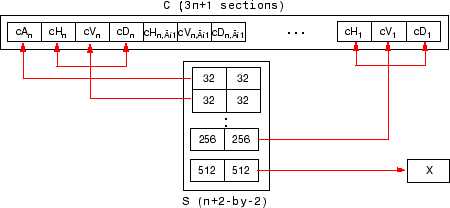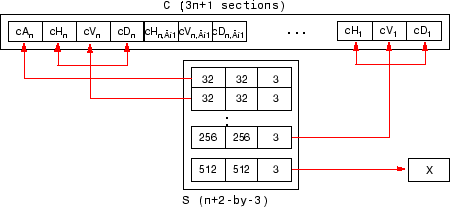# wthcoef2

2-D wavelet coefficient thresholding

## Syntax

``NC = wthcoef2('type',C,S,N,T,SORH)``
``NC = wthcoef2('type',C,S,N)``
``NC = wthcoef2('a',C,S)``
``NC = wthcoef2('t',C,S,N,T,SORH)``

## Description

example

````NC = wthcoef2('type',C,S,N,T,SORH)` returns the horizontal, vertical, or diagonal coefficients obtained from the wavelet decomposition structure `[C,S]` by soft or hard thresholding defined in vectors `N` and `T`. `wthcoef2` is a two-dimensional denoising and compression oriented function.```
````NC = wthcoef2('type',C,S,N)` returns the horizontal, vertical, or diagonal coefficients obtained from `[C,S]` by setting all the coefficients of detail levels defined in `N` to zero.```
````NC = wthcoef2('a',C,S)` returns the coefficients obtained by setting approximation coefficients to zero.```
````NC = wthcoef2('t',C,S,N,T,SORH)` returns the detail coefficients obtained from the wavelet decomposition structure `[C,S]` by soft or hard thresholding defined in vectors `N` and `T`. `[NC,S]` is the modified wavelet decomposition structure.```

## Examples

collapse all

`load mask`

Perform a level 2 wavelet decomposition of the image using the `haar` wavelet.

`[C,S]=wavedec2(X,2,'haar');`

Calculate the vertical coefficients obtained from the wavelet decomposition structure by soft thresholding defined in thresholding vectors `[1 2]` and `[2 4]`.

`NC = wthcoef2('v',C,S,[1 2],[2 4],'s')`
```NC = 1×65536 103 × 0.9280 0.9265 0.9295 0.9258 0.9305 0.9245 0.9340 0.9235 0.9268 0.9233 0.9213 0.9260 0.9220 0.9208 0.9203 0.9223 0.9278 0.9220 0.9265 0.9160 0.9290 0.9210 0.9280 0.9190 0.9280 0.9220 0.9303 0.9190 0.7363 0.7210 0.9218 0.9280 0.9330 0.9248 0.9308 0.9263 0.9285 0.9245 0.9330 0.9320 0.9340 0.9283 0.9283 0.9280 0.9328 0.9295 0.9228 0.9218 0.9340 0.9300 ```

## Input Arguments

collapse all

Type of coefficients obtained from the wavelet decomposition structure, specified as one of the following:

• `'h'`— Horizontal coefficients

• `'v'`— Vertical coefficients

• `'d'`— Diagonal coefficients

For more information, see `wthresh`.

Wavelet decomposition vector. The vector `C` contains the approximation and detail coefficients organized by level. The function uses the bookkeeping matrix `S` to parse `C`.

The vector `C` is organized as A(`N`), H(`N`), V(`N`), D(`N`), H(`N`-1), V(`N`-1), D(`N`-1), …, H(1), V(1), D(1), where A, H, V, and D are each a row vector. Each vector is the column-wise storage of a matrix.

• A contains the approximation coefficients.

• H contains the horizontal detail coefficients.

• V contains the vertical detail coefficients.

• D contains the diagonal detail coefficients.

For more information, see `wavedec2`.

Bookkeeping matrix. The matrix `S` contains the dimensions of the wavelet coefficients by level and the function uses it to parse the wavelet decomposition vector `C`.

• `S(1,:)` = size of approximation coefficients(`N`).

• `S(i,:)` = size of detail coefficients(`N-i+2`) for `i` = 2, ...`N+1` and `S(N+2,:) = size(X)`.

The following diagram shows the relationship between `C` and `S` in the wavelet decomposition of a 512-by-512 matrix.When `X` represents an indexed image, the output arrays cA, cH, cV, and cD are m-by-n matrices. When `X` represents a truecolor image, it is an m-by-n-by-3 array, where each m-by-n matrix represents a red, green, or blue color plane concatenated along the third dimension. The size of vector `C` and the size of matrix `S` depend on the type of the analyzed image.

For a truecolor image, the decomposition vector `C` and the corresponding bookkeeping matrix `S` can be represented as shown.For more information, see `wavedec2`.

Threshold vector, specified by a size `1 ```` N(i) ```` size(S,1)-2`. `N` contains the detail levels to be thresholded and `T` the corresponding thresholds.

Threshold vector, specified as a nonnegative vector.``` N``` and `T` must be of the same length. `N` contains the detail levels to be thresholded and `T` the corresponding thresholds.

Soft or hard threshold, specified as `'s'` or `'h'`.

For more information, see `wthresh`.

## Output Arguments

collapse all

Wavelet coefficient threshold, returned as a real-valued vector.

## Extended Capabilities

### C/C++ Code GenerationGenerate C and C++ code using MATLAB® Coder™.